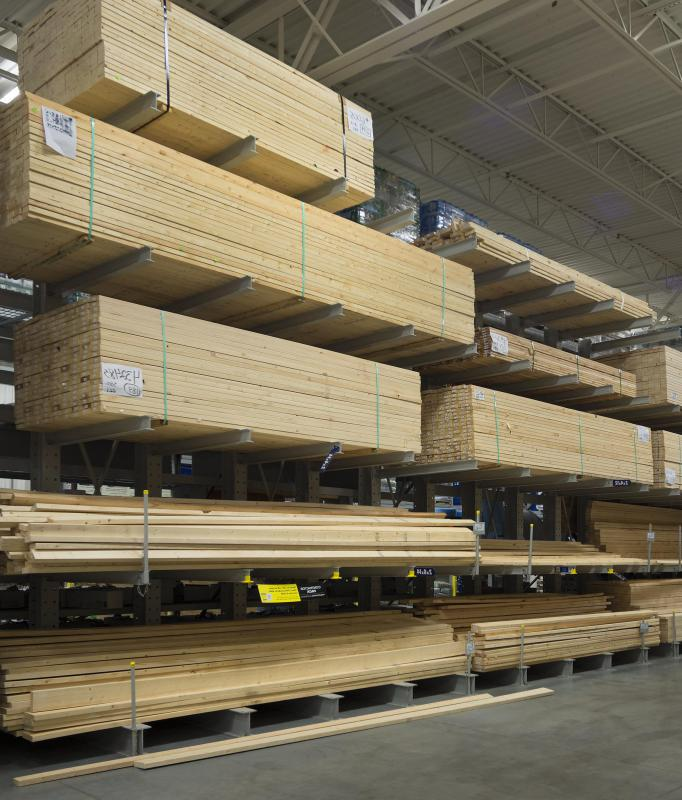# What Is a Linear Meter?

Christian Petersen
Christian PetersenThe term "linear meter" may be used when discussing amounts of a material, such as lumber.

A linear meter is the somewhat redundant term for a International System of Units (SI) measurement of length. A linear meter is different than a square meter which is a unit of area or a cubic meter which is a unit of volume. Generally, the word linear is omitted as it is understood that the meter is a unit of length rather than area or volume. Nonetheless, in some cases, it is written as "linear meter" to avoid confusion when multiple units are being used in a particular work or problem.

The metric system, which is the basis for the modern SI system, derives its name from the meter, which is one of the seven basic units of the SI system. The meter is defined as the distance that light travels in vacuum over a span of 1/299,792,458 of a second. This definition has evolved since its first official designation by the French Academy of Sciences in 1791, at which time it was described as one ten millionth of the distance from the North pole to the equator.

This initial definition of the meter was later deemed to have a margin of error which amounted to 0.2 mm (0.008 inches) due to a failure to account for the distortion in the Earth's spherical shape due to centrifugal forces associated with rotation which cause the Earth to bulge slightly at the equator and flatten slightly at the poles. Later, a new prototype for the meter, using the previous definition as a basis was forged from a platinum and iridium alloy, and kept under controlled conditions.

In 1960, the definition was changed yet again to further increase the precision of the linear meter. A wavelength of a certain type of radiation from decaying isotopes of the element krypton was used to define the meter. The current definition based on the transit time of light in a vacuum was adopted in 1983. All of these definitions did little to change the everyday usage of the linear meter by most common citizens. These definitions have always been more for the benefit of scientists and those who require extreme precision in measurements.

Today, the meter is one of the most recognizable units of measurement, even for countries such as the United States that have been slow to adopt the metric system. It is equivalent to a little more than 3.28 feet or 1.09 yards. One thousand meters is a kilometer, the standard unit used for larger distances in many parts of the world.

In some cases, the term linear meter is used when discussing amounts of a material such as lumber. In these cases, it is sometimes confused with square or cubic meters, units of area and volume. To avoid confusion, a linear meter must always be regarded as simply a measurement of length. When discussing lumber for example, a linear meter means a piece one meter long, regardless of the other dimensions of the board.

## You might also Likesdailey

Also, since "linear" has the word "line" in it, this should help someone know that this is referring to a length when using the term "linear meter."

Ledgenderous

It is easy to tell when someone is referring to a linear meter over a square or cubic meter when they are using abbreviations though. A m is used for linear meter, while a square meter is m2 and a cubic meter is m3.